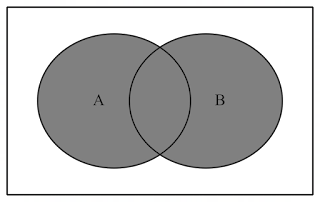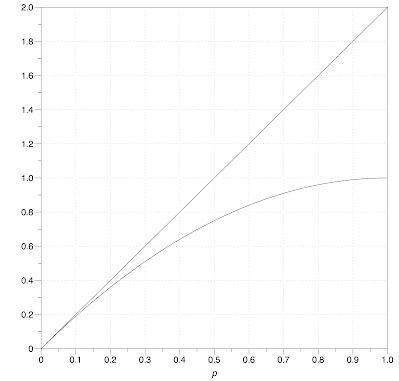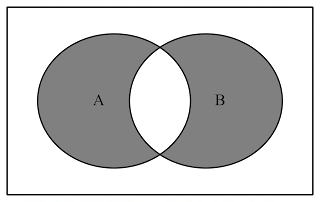# Colin Foster's Mathematics Education Blog

## 26 May 2022

### Are two cars better than one?

So many ideas in probability are really quite unintuitive. How can we help learners make better sense of how simple probabilities combine, without relying on arbitrary rules and mysterious formulae?

I recently heard someone talking about whether they should get a second car for their household (Note 1). They were doubtful that it was a good idea. In addition to the cost and environmental impact, they said, “If you have two cars, there’s twice as much chance that something will go wrong with one of them.” This seemed self-evidently true, and everybody nodded sadly, and the conversation moved on.

I began thinking about how I might respond mathematically. I knew what they meant, and their statement might indeed be approximately true, but it couldn’t be exactly true. Even if all that you know about probabilities is that they are capped at 1 (i.e., 100% is the highest possible probability), it is clear that you cannot just go around doubling probabilities. Doubling any probability greater than 0.5 will give you a total probability greater than 1, which is impossible. And, even if you have super-reliable cars with a very small probability $p$ of failing, you would only need to ensure that you buy more than $\frac{1}{p}$ of them for the total probability to exceed 1.

So, why is such a plausible-sounding statement not right? And under what assumptions might the statement be approximately true?

Suppose that both cars have the same probably $p$ of ‘something going wrong with them’ in a certain time interval. Would these be independent events? If both cars are parked outside the same house, then they are likely to be subject to similar weather conditions and other factors, so it seems unlikely that failure of one would be completely unrelated to failure of the other. But let’s ignore this and suppose that the two events are independent, and also that the two cars are equally likely to fail. This would mean that the probability of both cars failing would be $p^2$. And this means that when we double $p$ we are overcounting by $p^2$ (see Figure 1), because we are counting the same situation of ‘something goes wrong’ when car A fails and counting it again when car B fails, for those occasions when they both fail. In the extreme case, where you had two completely useless cars, you would have a 100% chance of not being able to drive anywhere, but not a 200% chance! Now, if $p$ is very small, then $p^2$ will be very very small, and so we can perhaps ignore the overlap region. But, if $p$ is not very small, then $p^2$ will be non-negligible. This means that the correct probability for either (or both) cars failing is $2p-p^2$.Figure 1. $p(A∪B)$

Looking at the expression $2p-p^2$, we might wonder whether we can be absolutely sure that it is always less than 1 for all values of $p$. Is the $p^2$ definitely always sufficiently large to bring back $2p$ to below 1 whenever $p>0.5$? One way to see this is by completing the square, to obtain $1-(1-p)^2$, meaning that $2p-p^2$ is equal to '$1-$something that is never negative'. Figure 2 shows the graph $y=2p-p^2=p(2-p)$, and the curve has its maximum value of 1 at $p=1$, and so it never exceeds 1 for any value of $p$ (Note 2).Figure 2. $y=2p$ and $y=2p-p^2$

We can also see in Figure 2 that the line $y=2p$ is indeed a good approximation to the curve for small values of $p$. So, just doubling the probability is a reasonable approximation if you are considering very reliable cars.

Using the algebra, this reasoning is quite straightforward for anyone comfortable with quadratics and elementary probability. In probability, we can only add the probability of events A and B if they are mutually exclusive (i.e., $p(A∩B)=0$), so that the ‘Venn diagram identity’ $p(A∪B)\equiv p(A)+p(B)-p(A∩B)$ reduces to $p(A∪B)=p(A)+p(B)$. In other cases, we have to subtract the intersection, so as not to double count it.

I was happy with all of this, but I wanted to say something that didn't sound technical or rely on set theory or even Venn diagrams. Could I say in words why doubling was not quite right? I found it hard to come up with a good way to explain to my friend why the possibility of both cars going wrong was relevant to their statement, and why this indeed made their statement technically wrong, even if you were willing to make assumptions about things like independence and so on. If I had said that having two cars that might go wrong is not quite twice as bad as having one car that might go wrong, because, at least some times, both cars will simultaneously go wrong, I think they would be quite surprised! The commonsense response is that there is no consolation in having both cars fail on the same day - that is the worst possible nightmare, and indeed one of the reasons for contemplating having a second car was to try to be sure that they would always have one working car! They would probably respond that “When I said 'either-or', I was including 'both'!”, which misses the point. Yes, we want to include the chance of both cars failing - the point is that we want to include that possibility only once, not twice (Figure 3)! It is still true that $p^2<p$, and possibly dramatically so ($p^2 \ll p$), so the chance of having at least one working car has indeed risen from $1-p$ to $1-p^2$. The point is that if last week Car A failed, say, on Monday, Thursday and Saturday, whereas Car B failed on Tuesday, Saturday and Sunday, our daily frequency of car trouble would have been $\frac{5}{7}$ and not $\frac{6}{7}$, because we don't double count Saturday just because it was a double-failure day.Figure 3. $(A∪B)-(A∩B)$

Perhaps this is part of a broader theme in mathematics of situations in which things can’t be simplistically added up. Other examples could include vectors that are not in the same direction, numerators of fractions that have different denominators, and dimensionally-incompatible quantities, such as distance and time (Foster, 2019). However, there is something particular about probability for me. I enjoy probability very much, but I think it's the area of mathematics in which I'm most likely to struggle to truly make sense or to be able to explain concepts clearly to others without using technical language and symbols (see Foster, 2021). Rarely when I'm doing a probability calculation do I have a rough ballpark estimate of the answer I should be getting, and, if I obtain an answer like $\frac{127}{351}$, it would be scarcely worth my while converting it to a decimal to see if it looked a 'reasonable' size, as I would have no idea how to tell reasonable from unreasonable. Do other people share this sense?

### Questions to reflect on

1. Do you have a better way of explaining why doubling is invalid here?

2. Do you have other examples of 'similar' situations to this?

3. Do you share my sense that probability is often 'harder to explain' than other areas of mathematics?

### Notes

1. With apologies for the highly 'middle-class' nature of this 'first-world problem'!

2. The expression $1-(1-p)^2$ can also be obtained intuitively by saying that the required probability is the complement of the probability that both cars are working properly. Since the probability that either car is working properly is $1-p$, the probability that both (assumed independent) work properly is $(1-p)^2$, and so the probability that this is not the case must be $1-(1-p)^2$.

### References

Foster, C. (2019). Questions pupils ask: Why can’t it be distance plus time? Mathematics in School, 48(1), 15–17. https://www.foster77.co.uk/Foster,%20Mathematics%20in%20School,%20Why%20can't%20it%20be%20distance%20plus%20time.pdf

Foster, C. (2021). In a spin. Teach Secondary, 10(1), 11. https://www.foster77.co.uk/Foster,%20Teach%20Secondary,%20In%20a%20spin.pdf

1.Very nice - I wonder if the sentiment/intuition of 'twice as much chance to go wrong' is really about expected values rather than probability - so really it's expressing that 2 cars may have twice as many total problems to deal with (still assuming the first car doesn't get used less etc.).

2.I am sure KO is right - the expected cost per year of repairs doubles (assuming identical cars!). I think expectations work more intuitively than probabilities, mainly because the expected value of the two RVs is the sum of the two expectations, even if the RVs are not independent.

### Teaching specific tactics for problem solving

This is my final blogpost, as my year as President of the Mathematical Association draws to a close, so I've allowed myself to go on at ...